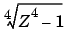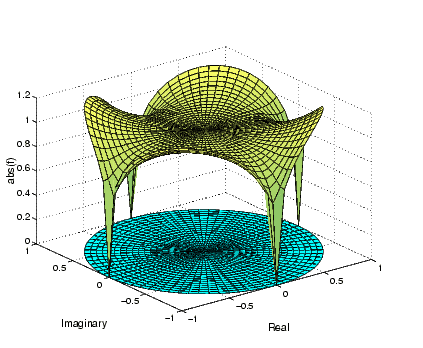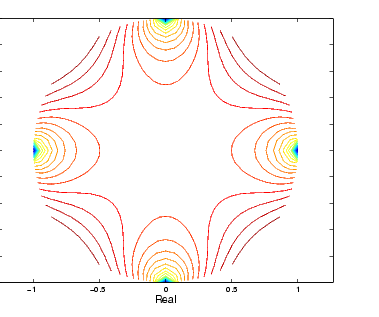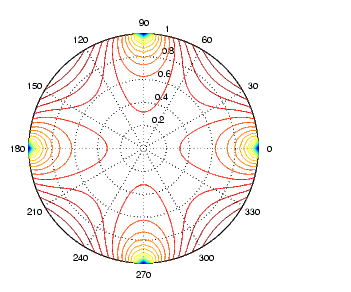GraphicsDisplaying Contours in Polar Coordinates

You can contour data defined in the polar coordinate system. As an example, set up a grid in polar coordinates and convert the coordinates to Cartesian coordinates.

• ```[th,r] = meshgrid((0:5:360)*pi/180,0:.05:1);
[X,Y] = pol2cart(th,r);
```

Then generate the complex matrix `Z` on the interior of the unit circle.

• ```Z = X+i*Y;
```

`X`, `Y`, and `Z` are points inside the circle.

Create and display a surface of the function.

• ```f = (Z.^4-1).^(1/4);
surf(X,Y,abs(f))
```

Display the unit circle beneath the surface using the statements

• ```hold on
surf(X,Y,zeros(size(X)))
hold off```

Labeling the Graph

These statements add labels.

• ````xlabel('Real',`'FontSize',14```);
```ylabel('Imaginary','FontSize',14);
zlabel('abs(f)','FontSize',14);
```

Contours in Cartesian Coordinates

These statements display a contour of the surface in Cartesian coordinates and label the x- and y-axis.

• ```contour(X,Y,abs(f),30)
axis equal
`xlabel('Real',`'FontSize',14```);
```ylabel('Imaginary','FontSize',14);```

Contours on a Polar Axis

You can also display the contour within a polar axes. Create a polar axes using the `polar` function, and then delete the line specified with `polar`.

• ```h = polar([0 2*pi], [0 1]);
delete(h)
```

With `hold` `on`, display the contour on the polar grid.

• ```hold on
contour(X,Y,abs(f),30)```Changing the Offset of a Contour Interactive Plotting© 1994-2005 The MathWorks, Inc.